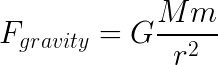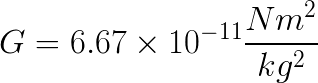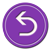# National Aeronautics and Space Administration

## Goddard Space Flight Center## More on Newton's Law of Universal Gravitation

### More on Newton's Law of Universal GravitationPrehistoric man realized a long time ago that when objects are released near the surface of the Earth, they always fall down to the ground. In other words, the Earth attracts objects near its surface to itself.

Galileo (1564-1642) pointed out that heavy and light objects fall toward the earth at the same rate (so long as air resistance is the same for each). But it took Sir Isaac Newton (in 1666) to realize that this force of attraction between masses is universal!

Newton proved that the force that causes, for example, an apple to fall toward the ground is the same force that causes the moon to fall around, or orbit, the Earth. This universal force also acts between the Earth and the Sun, or any other star and its satellites. Each attracts the other.

Sir Isaac Newton defined this attraction mathematically. The force of attraction between two masses is directly proportional to the product of their masses and inversely proportional to the square of the distance between their centers. This is all multiplied by a universal constant whose value was determined by Henry Cavendish in 1798.Where G is the gravitational constant, M is one mass, m is the second mass, and r is the distance between the two masses. The value of the universal gravitational constant, G, is:A service of the High Energy Astrophysics Science Archive Research Center (HEASARC), Dr. Alan Smale (Director), within the Astrophysics Science Division (ASD) at NASA/GSFC